include/glu3.h File Reference

#include <GL/gl.h>
#include <GL/glext.h>
#include "glu3_scalar.h"

Go to the source code of this file.

Classes

struct  GLUvec4
struct  GLUmat4
struct  GLUmat4Stack
struct  GLUarcball
class  GLUshapeConsumer
class  GLUshapeProducer
class  GLUsphereProducer
class  GLUcubeProducer

Defines

#define GLU3_VERSION_0_1
#define GLU_MAX_STACK_DEPTH   32

Functions

GLUvec4 operator* (GLfloat f, const GLUvec4 &v)
GLUvec4operator+= (GLUvec4 &l, const GLUvec4 &r)
GLUvec4operator-= (GLUvec4 &l, const GLUvec4 &r)
GLUvec4operator*= (GLUvec4 &l, const GLUvec4 &r)
GLUvec4operator*= (GLUvec4 &l, GLfloat r)
GLfloat gluDot4_4v (const GLUvec4 *, const GLUvec4 *)
GLfloat gluDot3_4v (const GLUvec4 *, const GLUvec4 *)
GLfloat gluDot2_4v (const GLUvec4 *, const GLUvec4 *)
void gluCross4v (GLUvec4 *result, const GLUvec4 *u, const GLUvec4 *v)
void gluNormalize4v (GLUvec4 *result, const GLUvec4 *u)
GLfloat gluLength4v (const GLUvec4 *u)
GLfloat gluLengthSqr4v (const GLUvec4 *)
void gluOuter4v (GLUmat4 *result, const GLUvec4 *u, const GLUvec4 *v)
void gluMult4v_4v (GLUvec4 *result, const GLUvec4 *, const GLUvec4 *)
void gluDiv4v_4v (GLUvec4 *result, const GLUvec4 *, const GLUvec4 *)
void gluAdd4v_4v (GLUvec4 *result, const GLUvec4 *, const GLUvec4 *)
void gluSub4v_4v (GLUvec4 *result, const GLUvec4 *, const GLUvec4 *)
void gluMult4v_f (GLUvec4 *result, const GLUvec4 *, GLfloat)
void gluDiv4v_f (GLUvec4 *result, const GLUvec4 *, GLfloat)
void gluAdd4v_f (GLUvec4 *result, const GLUvec4 *, GLfloat)
void gluSub4v_f (GLUvec4 *result, const GLUvec4 *, GLfloat)
void gluMult4m_4m (GLUmat4 *result, const GLUmat4 *, const GLUmat4 *)
void gluAdd4m_4m (GLUmat4 *result, const GLUmat4 *, const GLUmat4 *)
void gluSub4m_4m (GLUmat4 *result, const GLUmat4 *, const GLUmat4 *)
void gluMult4m_4v (GLUvec4 *result, const GLUmat4 *m, const GLUvec4 *v)
void gluMult4m_f (GLUmat4 *result, const GLUmat4 *, GLfloat)
void gluScale4v (GLUmat4 *result, const GLUvec4 *u)
void gluRotate4v (GLUmat4 *result, const GLUvec4 *axis, GLfloat angle)
void gluLookAt4v (GLUmat4 *result, const GLUvec4 *eye, const GLUvec4 *center, const GLUvec4 *up)
void gluTranspose4m (GLUmat4 *result, const GLUmat4 *m)
GLfloat gluDeterminant4_4m (const GLUmat4 *m)
GLboolean gluInverse4_4m (GLUmat4 *result, const GLUmat4 *m)
const GLchar * gluLoadTextFile (const char *file_name)
void gluUnloadTextFile (const GLchar *text)
void gluArcballViewport (GLUarcball *ball, unsigned x, unsigned y, unsigned width, unsigned height)
void gluArcballClick (GLUarcball *ball, unsigned start_x, unsigned start_y)
void gluArcballDrag (GLUarcball *ball, GLUmat4 *transformation, unsigned end_x, unsigned end_y)
GLfloat gluDot4 (const GLUvec4 &, const GLUvec4 &)
GLfloat gluDot3 (const GLUvec4 &, const GLUvec4 &)
GLfloat gluDot2 (const GLUvec4 &, const GLUvec4 &)
GLUvec4 gluCross (const GLUvec4 &u, const GLUvec4 &v)
GLUvec4 gluNormalize (const GLUvec4 &v)
GLfloat gluLength (const GLUvec4 &u)
GLfloat gluLengthSqr (const GLUvec4 &u)
GLUmat4 gluScale (const GLUvec4 &u)
GLUmat4 gluScale (GLfloat x, GLfloat y, GLfloat z)
GLUmat4 gluRotate (const GLUvec4 &axis, GLfloat angle)
GLUmat4 gluLookAt (const GLUvec4 &eye, const GLUvec4 &center, const GLUvec4 &up)
GLfloat gluDeterminant4 (const GLUmat4 &m)
GLboolean gluInverse4 (GLUmat4 &result, const GLUmat4 &m)
GLUmat4 gluInverse4 (const GLUmat4 &m)
Translation matrix

void gluTranslate3f (GLUmat4 *result, GLfloat x, GLfloat y, GLfloat z)
void gluTranslate4v (GLUmat4 *result, const GLUvec4 *v)
GLUmat4 gluTranslate (GLfloat x, GLfloat y, GLfloat z)
GLUmat4 gluTranslate (const GLUvec4 &v)
Projection matrix

Functions that generate various common projection matrixes.

void gluFrustum6f (GLUmat4 *result, GLfloat left, GLfloat right, GLfloat bottom, GLfloat top, GLfloat near, GLfloat far)
void gluPerspective4f (GLUmat4 *result, GLfloat fovy, GLfloat aspect, GLfloat near, GLfloat far)
void gluOrtho4f (GLUmat4 *result, GLfloat left, GLfloat right, GLfloat bottom, GLfloat top)
void gluOrtho6f (GLUmat4 *result, GLfloat left, GLfloat right, GLfloat bottom, GLfloat top, GLfloat near, GLfloat far)

Variables

const GLUmat4 gluIdentityMatrix

Detailed Description

Interface definitions for GLU3 library.

Function Documentation

 void gluAdd4m_4m ( GLUmat4 * result, const GLUmat4 * , const GLUmat4 * )

Component-wise addition with a mat4.

GLUmat4::operator+
 void gluAdd4v_4v ( GLUvec4 * result, const GLUvec4 * , const GLUvec4 * )

Component-wise add two vec4s

GLUvec4::operator+
 void gluAdd4v_f ( GLUvec4 * result, const GLUvec4 * , GLfloat )

Add a scalar to each of the components of a vector.

 GLUvec4 gluCross ( const GLUvec4 & u, const GLUvec4 & v ) [inline]

Cross product from vec4 sources

The 3-dimensional cross product of u and v is calculated. The result is stored in the first three components of result. The fourth component is set to 0.0.

gluCross4v
 void gluCross4v ( GLUvec4 * result, const GLUvec4 * u, const GLUvec4 * v )

Cross product from vec4 sources

The 3-dimensional cross product of u and v is calculated. The result is stored in the first three components of result. The fourth component is set to 0.0.

gluCross (C++)
 GLfloat gluDeterminant4 ( const GLUmat4 & m ) [inline]

Calculate the determinant of a matrix.

gluDeterminant4_4m
 GLfloat gluDeterminant4_4m ( const GLUmat4 * m )

Calculate the determinant of a matrix.

gluDeterminant4 (C++)
 void gluDiv4v_4v ( GLUvec4 * result, const GLUvec4 * , const GLUvec4 * )

Component-wise divide two vec4s

 void gluDiv4v_f ( GLUvec4 * result, const GLUvec4 * , GLfloat )

Divide components of a vector by a scalar.

 GLfloat gluDot2 ( const GLUvec4 & , const GLUvec4 & )

Two component dot product from vec4 sources.

gluDot2_4v
 GLfloat gluDot2_4v ( const GLUvec4 * , const GLUvec4 * )

Two component dot product from vec4 sources.

gluDot2 (C++)
 GLfloat gluDot3 ( const GLUvec4 & , const GLUvec4 & )

Three component dot product from vec4 sources.

gluDot3_4v
 GLfloat gluDot3_4v ( const GLUvec4 * , const GLUvec4 * )

Three component dot product from vec4 sources.

gluDot3 (C++)
 GLfloat gluDot4 ( const GLUvec4 & , const GLUvec4 & )

Four component dot product from vec4 sources.

gluDot4_4v
 GLfloat gluDot4_4v ( const GLUvec4 * , const GLUvec4 * )

Four component dot product from vec4 sources.

gluDot4 (C++)
 void gluFrustum6f ( GLUmat4 * result, GLfloat left, GLfloat right, GLfloat bottom, GLfloat top, GLfloat near, GLfloat far )

Generate a perspective projection matrix

Parameters:
 result Location to store the calculated matrix left Coordinate for the left clipping plane right Coordinate for the right clipping plane top Coordinate for the top clipping plane bottom Coordinate for the bottom clipping plane near Distance to the near plane far Distance to the far plane

The matrix calculated is: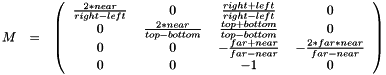If left = right, top = bottom, or near = far, the function returns without writing any value to result.

If eithernear or far are negative, the function returns without writing any value to result.

 GLUmat4 gluInverse4 ( const GLUmat4 & m ) [inline]

Calculate the inverse of a matrix.

Returns:
The inverse of the matrix m. If m is not invertable, the return result is undefined.
Warning:
This function is really only safe when the input matrix is known to be invertable. Nearly all well behaved transformation matrices fall into this category.
gluInverse4_4m
 GLboolean gluInverse4 ( GLUmat4 & result, const GLUmat4 & m ) [inline]

Calculate the inverse of a matrix.

Inverts the matrix m and stores the result in result. If m is not invertable, result is not modified

Returns:
If the matrix is invertable (i.e., the determinant is not zero), GL_TRUE is returned. Otherwise GL_FALSE is returned.
gluInverse4_4m
 GLboolean gluInverse4_4m ( GLUmat4 * result, const GLUmat4 * m )

Calculate the inverse of a matrix.

Inverts the matrix m and stores the result in result. If m is not invertable, result is not modified

Returns:
If the matrix is invertable (i.e., the determinant is not zero), GL_TRUE is returned. Otherwise GL_FALSE is returned.
gluInverse4 (C++)
 GLfloat gluLength ( const GLUvec4 & u ) [inline]

Calculate the length of a vec4

The length (magnitude) the 4-dimensional vector u is returned.

gluLength4v
 GLfloat gluLength4v ( const GLUvec4 * u )

Calculate the length of a vec4

The length (magnitude) the 4-dimensional vector u is returned.

gluLength (C++)
 GLfloat gluLengthSqr ( const GLUvec4 & u ) [inline]

Calculate the squared length of a vec4

The squared length (magnitude) the 4-dimensional vector u is returned.

gluLengthSqr4v
 GLfloat gluLengthSqr4v ( const GLUvec4 * )

Calculate the squared length of a vec4

The squared length (magnitude) the 4-dimensional vector u is returned.

gluLengthSqr (C++)
 const GLchar* gluLoadTextFile ( const char * file_name )

Load a text file from disk

Parameters:
 file_name Name of the file to be loaded

Loads data from a named text file and returns a pointer to that data to the caller. This may be useful, for example, for loading shader code from flies on disk.

The pointer returned by this function should later be released by calling gluUnloadTextFile.

Note:
The data pointed to by the return value if this function really is constant. On some systems this function may be implemented by creating a read-only mapping of the file. Writes to such data will result in program termination.
 GLUmat4 gluLookAt ( const GLUvec4 & eye, const GLUvec4 & center, const GLUvec4 & up ) [inline]

Calculate a viewing transformation

Parameters:
 eye Position, in 3-dimensional space, of the eye point. center Position, in 3-dimensional space, that the eye is looking at. up Direction of the up vector. result Storage for the resulting matrix.

Calculates a transformation matrix that maps the eye point to the origin and the center to the negative Z axis. The direction defined by up is projected onto the X/Y plane an is mapped to the positive Y axis.

The calculated matrix is: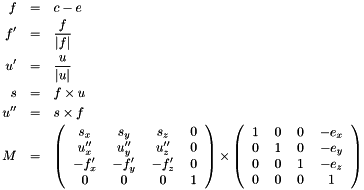gluLookAt4v
 void gluLookAt4v ( GLUmat4 * result, const GLUvec4 * eye, const GLUvec4 * center, const GLUvec4 * up )

Calculate a viewing transformation

Parameters:
 eye Position, in 3-dimensional space, of the eye point. center Position, in 3-dimensional space, that the eye is looking at. up Direction of the up vector. result Storage for the resulting matrix.

Calculates a transformation matrix that maps the eye point to the origin and the center to the negative Z axis. The direction defined by up is projected onto the X/Y plane an is mapped to the positive Y axis.

The calculated matrix is:gluLookAt (C++)
 void gluMult4m_4m ( GLUmat4 * result, const GLUmat4 * , const GLUmat4 * )

Matrix multiply with a 4x4 matrix.

GLUmat4::operator*
 void gluMult4m_4v ( GLUvec4 * result, const GLUmat4 * m, const GLUvec4 * v )

Multiply a vector with a matrix.

Multiply as a column-vector with a 4x4 matrix resulting in a column-vector.

GLUmat4::operator*
 void gluMult4m_f ( GLUmat4 * result, const GLUmat4 * , GLfloat )

Multiply each component of a matrix with a scalar

GLUmat4::operator*
 void gluMult4v_4v ( GLUvec4 * result, const GLUvec4 * , const GLUvec4 * )

Component-wise multiply two vec4s

GLUvec4::operator*
 void gluMult4v_f ( GLUvec4 * result, const GLUvec4 * , GLfloat )

Multiply with a scalar.

GLUvec4::operator*
 GLUvec4 gluNormalize ( const GLUvec4 & v ) [inline]

Normalize a vec4

The 4-dimensional normalization of u is stored in result.

gluNormalize4v
 void gluNormalize4v ( GLUvec4 * result, const GLUvec4 * u )

Normalize a vec4

The 4-dimensional normalization of u is stored in result.

gluNormalize (C++)
 void gluOrtho4f ( GLUmat4 * result, GLfloat left, GLfloat right, GLfloat bottom, GLfloat top )

Generate an orthographic projection matrix

Parameters:
 result Location to store the calculated matrix left Coordinate for the left clipping plane right Coordinate for the right clipping plane top Coordinate for the top clipping plane bottom Coordinate for the bottom clipping plane

The matrix calculated is:If left = right or top = bottom the function returns without writing any value to result.

This function is identical to calling gluOrtho6f with near = -1 and far = 1.

gluOrtho6f
 void gluOrtho6f ( GLUmat4 * result, GLfloat left, GLfloat right, GLfloat bottom, GLfloat top, GLfloat near, GLfloat far )

Generate an orthographic projection matrix

Parameters:
 result Location to store the calculated matrix left Coordinate for the left clipping plane right Coordinate for the right clipping plane top Coordinate for the top clipping plane bottom Coordinate for the bottom clipping plane near Distance to the near plane far Distance to the far plane

The matrix calculated is: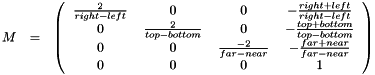If left = right, top = bottom, or near = far, the function returns without writing any value to result.

gluOrtho4f

 void gluOuter4v ( GLUmat4 * result, const GLUvec4 * u, const GLUvec4 * v )

Calculate the four dimensional outer product of two vec4 sources

Assuing u and v are column vectors, the outer product is: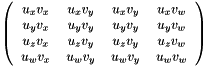void gluPerspective4f ( GLUmat4 * result, GLfloat fovy, GLfloat aspect, GLfloat near, GLfloat far )

Calculate a perspective projection matrix

Parameters:
 result Storage for the resulting matrix. fovy Field-of-view in the Y direction aspect The ratio of the size in the X direction to the size in the Y direction. This is used to calculate the field-of-view in the X direction. near Distance to the near plane far Distance to the far plane

The matrix calculated is: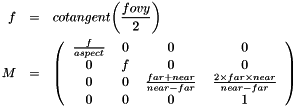GLUmat4 gluRotate ( const GLUvec4 & axis, GLfloat angle ) [inline]

Calculate a rotation matrix around an arbitrary axis

Parameters:
 axis Axis, based at the origin, around which to rotate angle Angle of rotation in degrees

If the specificed axis is not unit length, the vector will be normalized.

gluRotate4v
 void gluRotate4v ( GLUmat4 * result, const GLUvec4 * axis, GLfloat angle )

Calculate a rotation matrix around an arbitrary axis

Parameters:
 axis Axis, based at the origin, around which to rotate angle Angle of rotation in degrees

If the specificed axis is not unit length, the vector will be normalized.

gluRotate (C++)
 GLUmat4 gluScale ( GLfloat x, GLfloat y, GLfloat z ) [inline]

Calculate a scaling transformation matrix from a vector

A scaling transformation matrix is created using the x, y, and z components of u. Specifically, the matrix generated is: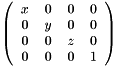gluScale4v
 GLUmat4 gluScale ( const GLUvec4 & u ) [inline]

Calculate a scaling transformation matrix from a vector

A scaling transformation matrix is created using the x, y, and z components of u. Specifically, the matrix generated is: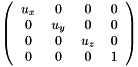gluScale4v
 void gluScale4v ( GLUmat4 * result, const GLUvec4 * u )

Calculate a scaling transformation matrix from a vector

A scaling transformation matrix is created using the x, y, and z components of u. Specifically, the matrix generated is:gluScale (C++)
 void gluSub4m_4m ( GLUmat4 * result, const GLUmat4 * , const GLUmat4 * )

Component-wise subtraction with a mat4.

GLUmat4::operator-
 void gluSub4v_4v ( GLUvec4 * result, const GLUvec4 * , const GLUvec4 * )

Component-wise subtract two vec4s

GLUvec4::operator-
 void gluSub4v_f ( GLUvec4 * result, const GLUvec4 * , GLfloat )

Subtract a scalar from each of the components of a vector.

 GLUmat4 gluTranslate ( const GLUvec4 & v ) [inline]

Calculate a translation matrix using components of a vector

The matrix generated is: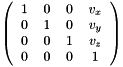gluTranslate4v
 GLUmat4 gluTranslate ( GLfloat x, GLfloat y, GLfloat z ) [inline]

Calculate a translation matrix using x, y, and z offsets

The matrix generated is:gluTranslate3f
 void gluTranslate3f ( GLUmat4 * result, GLfloat x, GLfloat y, GLfloat z )

Calculate a translation matrix using x, y, and z offsets

The matrix generated is:gluTranslate (C++)
 void gluTranslate4v ( GLUmat4 * result, const GLUvec4 * v )

Calculate a translation matrix using components of a vector

The matrix generated is:gluTranslate (C++)
 void gluTranspose4m ( GLUmat4 * result, const GLUmat4 * m )

Calculate the transpose of a matrix.

 void gluUnloadTextFile ( const GLchar * text )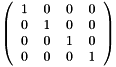Generated by1.6.2